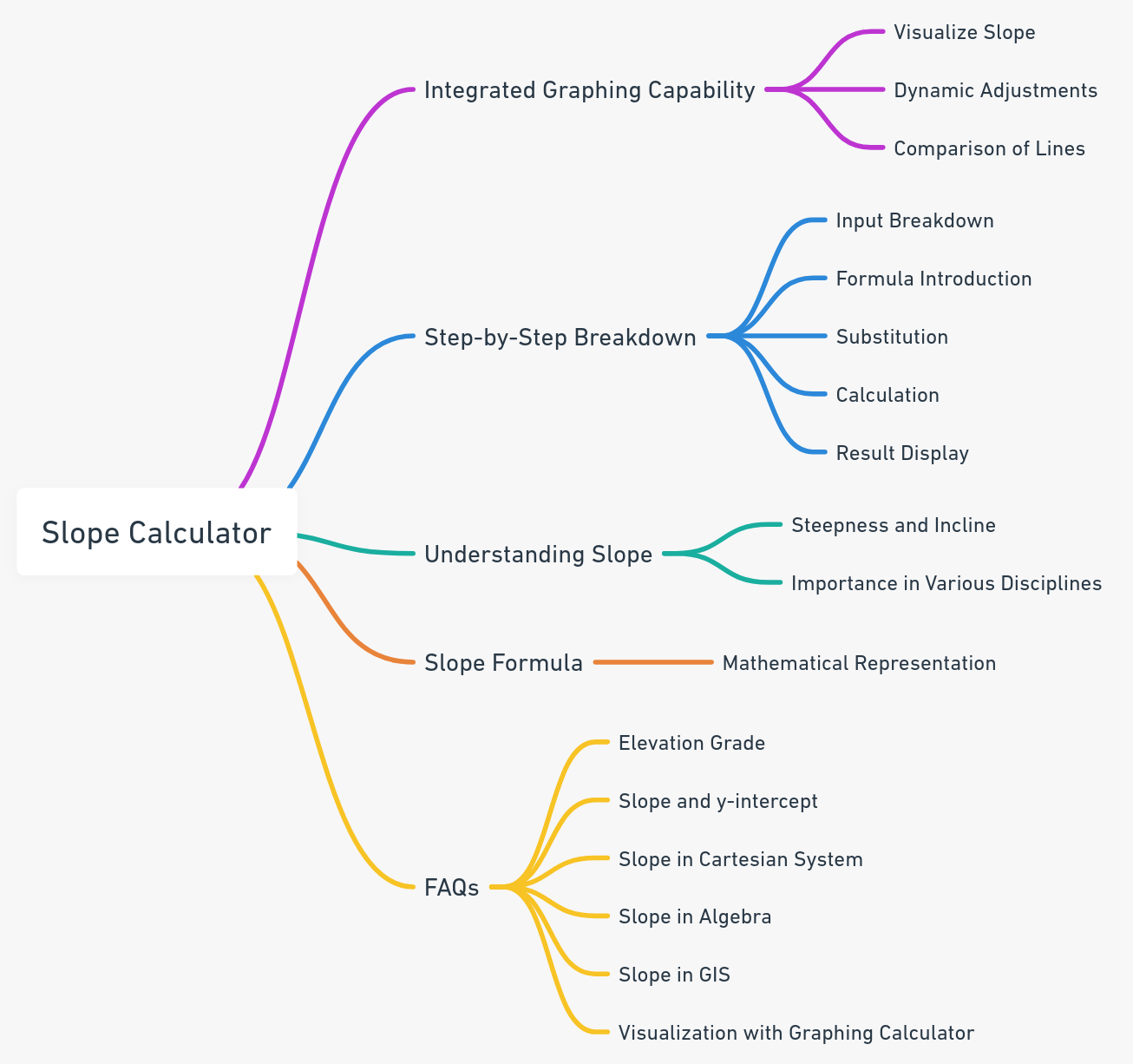Even The mini Tools Can Empower People to Do Great Things.

# Slope Calculator: Simplifying the Complexities of Line Inclines

Slope Calculator
Enter two points (x1, y1) and (x2, y2):
 x1: y1: x2: y2:

Embed Slope Calculator WidgetThe Slope Calculator is an invaluable tool designed to assist users in determining the slope or gradient of a line passing through two distinct points.

One of the standout features of our Slope Calculator is the integrated graphing capability. This feature allows users to not only calculate the slope but also to visualize it, offering a more comprehensive understanding of the concept.

Our Slope Calculator also goes beyond just providing a result. It offers a step-by-step breakdown of the entire calculation process, ensuring users grasp the underlying mathematics.

## Understanding the Concept of Slope

The slope of a line represents its steepness or incline. A line with a pronounced slope value is steeper, while one with a value closer to zero is relatively flat. The slope is integral in various disciplines, including engineering, architecture, and geography, as it aids in comprehending the orientation and behavior of linear structures and terrains.

## The Formula for Slope

The slope m of the line through any two points (x1, y1) and (x2, y2) is given by:## FAQ

Elevation grade provides insights into the steepness or incline of a surface. In slope calculation, it helps determine the angle of elevation from the horizontal plane, making it crucial for construction, engineering, and geographical studies.

For a linear equation in the form y = mx + b, 'm' represents the slope, and 'b' is the y-intercept. By rearranging the equation or using specific points, you can determine these values. You can also check our Slope Intercept Form (y=mx+b) Calculator.

In the Cartesian coordinate system, the slope represents the rate of change between the x and y coordinates. It indicates how much y changes for a unit change in x, helping in understanding the orientation and steepness of a line on the graph.

The formula is Slope (m) = (y2 - y1) / (x2 - x1), where (x1, y1) and (x2, y2) are two distinct points on the line.

In GIS, the slope can be calculated using digital elevation models (DEMs). By analyzing the elevation values of specific points, the software can compute the slope between them.

Input the equation or points into the online graphing calculator. It will plot the line and provide a visual representation, helping in understanding the slope and other related parameters.

## Reference

Reference this content, page, or tool as:

"Slope Calculator" at https://miniwebtool.com/slope-calculator/ from miniwebtool, https://miniwebtool.com/

by miniwebtool team. Updated: Oct 04, 2023